# Exponential Deviation Bands

The importable AIQ EDS file based on Vitali Apirine’s article in July 2019 Stocks & Commodities issue, “Exponential Deviation Bands,”

and a recreated Excel spreadsheet similar to the one shown in the article can be obtained on request via email to info@TradersEdgeSystems.com. The code is also shown here:

Exponential deviation (ED) bands are plotted above and below a moving average (MA) from which the bands are calculated. An exponential deviation from the moving average is used to set the bands. ED bands can be used with either a simple moving average (SMA) or an exponential moving average (EMA). The moving average dictates direction, and the exponential deviation sets band width. Breakouts from the band and changes in the band’s direction can help identify price trends and price reversals. These bands can be used on a variety of securities with its standard settings.

```!EXPONENTIAL DEVIATION BANDS
!Author: Vitali Apirine, TASC July 2019
!Coded by: Richard Denning, 5/15/2019

C is [close].
Periods is 20.

MA20 is simpleavg(C,Periods).   !expavg(C,Periods).  !or simpleavg(C,Periods).
MDev20 is (Abs(MA20-C)+Abs(MA20-valresult(C,1))+Abs(MA20-valresult(C,2))+Abs(MA20-valresult(C,3))
+Abs(MA20-valresult(C,4))+Abs(MA20-valresult(C,5))+Abs(MA20-valresult(C,6))+Abs(MA20-valresult(C,7))
+Abs(MA20-valresult(C,8))+Abs(MA20- valresult(C,9))+Abs(MA20- valresult(C,10))+Abs(MA20- valresult(C,11))
+Abs(MA20- valresult(C,12))+Abs(MA20- valresult(C,13))+Abs(MA20- valresult(C,14))+Abs(MA20- valresult(C,15))
+Abs(MA20- valresult(C,16)) +Abs(MA20- valresult(C,17))+Abs(MA20- valresult(C,18))+Abs(MA20- valresult(C,19)))/20.

Dev is Abs(MA20-C).
Rate is 2/( Periods +1).

DaysInto is ReportDate() - RuleDate().
Stop if DaysInto >= 200.
stopEXD is iff(Stop,Mdev20, EXD).
EXD is Dev*Rate + valresult(stopEXD,1)*(1-Rate).

UpperExp is MA20+2*EXD.
MidExp is MA20.
LowerExp is MA20-2*EXD.

ShowValues if 1.
```

Figure 9 shows the exponential deviation bands centered on a 20-bar simple moving average on a chart of the New York Composite Index (NYA).

FIGURE 9: AIQ. Here are exponential deviation bands centered on a 20-bar simple moving average on a chart of the New York Composite Index (NYA).

—Richard Denning
for AIQ Systems

# Backtesting A Mean-Reversion Strategy In Python

The importable AIQ EDS file based on Anthony Garner’s article in May 2019 Stocks & Commodities “Backtesting A Mean-Reversion Strategy In Python,” can be obtained on request via email to info@TradersEdgeSystems.com. The code is also shown below.

I backtested the author’s mean-reversion system (MeanRev.eds) using both the EDS module, which tests every trade on a one-share basis, and also via the Portfolio Manager, which performs a trading simulation.

The short side strategy showed a loss overall in the EDS test so I tested only the long side in the Portfolio Manager. I selected trades using the z-score, taking the lowest values.

For capitalization, I used max of three trades per day with a max total of 10 open trades at one time, 10% allocated to each position. I did not deduct slippage but did deduct commissions. I used a recent list of the NASDAQ 100 stocks to run the test. The equity curve and account statistics report are shown in Figure 7.

FIGURE 7: AIQ. This shows the equity curve (blue line) from long-only trading the NASDAQ 100 list of stocks from 1999 to March 15, 2019. The red line is the NDX index.

`!Backtesting a Mean-Reversion Strategy In Python !Author: Anthony Garner, TASC May 2019 !Coded by: Richard Denning 3/14/19 !www.TradersEdgeSystems.com !ABBREVIATIONS: C is [close]. !INPUTS: meanLen is 10. longZmult is -1. shortZmult is 1. meanMult is 10. !FORMULAS: SMA is simpleavg(C,meanLen). LMA is simpleavg(C,meanLen*meanMult). STD is sqrt(variance(C,meanLen)). zScore is (C - SMA) / STD.   !TRADING SIGNALS & EXITS: buyLong if zScore < longZmult and SMA > LMA. sellShort if zScore > shortZmult and SMA < LMA. exitLong if valresult(zScore,1) < -0.5 and zScore > 0.5. exitShort if valresult(zScore,1) > 0.5 and zScore < -0.5. showValues if 1. `

—Richard Denning
for AIQ Systems

# Weekly & Daily Stochastics

The AIQ code based on Vitali Apirine’s article in the September issue of Stocks and Commodities, “Weekly and Daily Stochastics, is provided below

Using Apirine’s weekly and daily stochastic indicators and a moving average to determine trend direction, I created an example system (long only) with the following rules:

Enter long next bar at open when all of the following are true:

1. The 200-day simple average of the NDX is greater than the day before
2. The 200-day simple average of the stock is greater than the day before
3. Both the weekly and daily stochastic indicators have been below 20 in the last five days
4. Both the weekly and daily stochastic indicators are greater than the day before.

I tested three exits. Figure 8 shows a 21-day hold then exit. Figure 9 shows a three-moving-average trend-following exit. Figure 10 shows an exit using only the weekly &amp; daily stochastic, once both are lower than the day before.FIGURE 8: AIQ, BUY and HOLD. Here is the sample equity curve (blue) compared to the NDX (red) for the test using a 21-day hold exit.FIGURE 9: AIQ, TREND-FOLLOWING EXIT. Here is the sample equity curve (blue) compared to the NDX index (red) for the test using a trend-following exit.FIGURE 10: AIQ, W and D STOCHASTIC EXIT. Here is the sample equity curve (blue) compared to the NDX index (red) for the test using the weekly and daily stochastic indicators.

The 21-day hold test showed a 11.2% return with a maximum drawdown of 29.3%. The trend-following exit test showed a 17.6% return with a maximum drawdown of 28.8%. The test using an exit based on only the weekly and daily stochastic indicators showed a return of 2.9% with a maximum drawdown of 32.5%. All the tests used the same entry rule and were run on an old 2016 list of the NASDAQ 100 stocks with the stocks that are no longer trading deleted.

```!WEEKLY AND DAILY STOCHASTIC
!Author: Vitali Apirine, TASC Sept 2018
!Coded by: Richard Denning 7/7/2018

!INPUTS:
Periods is 14.
Periods1 is 3.
Pds is 70.
Pds1 is 3.
smaLen1 is 70.
exitType is 1.

!ABBREVIATIONS:
C is [close].
H is [high].
L is [low].

!INDICATOR CODE:
STOCD is (C-LOWRESULT(L,Periods))/(HIGHRESULT(H,Periods)-LOWRESULT(L,Periods))*100.
SD is Simpleavg(Stocd,Periods1).
StocW is (C-LOWRESULT(L,Pds))/(HIGHRESULT(H,Pds)-LOWRESULT(L,Pds))*100.
SW is Simpleavg(Stocw,Pds1).
HD if hasdatafor(1000) &gt;= 500.
SMA200 is simpleavg(C,200).
SMA200ndx is tickerUDF("NDX",SMA200).

!SYSTEM CODE:
and SMA200 &gt; valresult(SMA200,1)
and SW &gt; valresult(SW,1)
and SD &gt; valresult(SD,1)
and countof(SW &lt; 20,5)&gt;=1
and countof(SD &lt; 20,5)&gt;=1
and HD.
smaLen2 is smaLen1*2.
smaLen3 is smaLen1*4.
SMA1 is simpleavg(C,smaLen1).
SMA2 is simpleavg(C,smaLen2).
SMA3 is simpleavg(C,smaLen3).
PD is {position days}.

!EXIT TYPE 1 USES THE INDICATOR ONLY
!EXIT TYPE 2 IS TREND FOLLOWING
Sell if (SD &lt; valresult(SD,1) and SW &lt; valresult(SW,1) and exitType=1)
or (exitType = 2
and ((Valresult(C,PD)valresult(SMA1,PD) And Cvalresult(SMA2,PD) And Cvalresult(SMA3,PD) And C 250)).

RSL is C/valresult(C,240).
```

—Richard Denning

for AIQ Systems

# Portfolio Strategy Based On Accumulation/Distribution

The AIQ code based on Domenico D’Errico’s article in the August issue of Stocks &amp; Commodities magazine, “Portfolio Strategy Based On Accumulation/Distribution,” is shown below.

“Whether you are an individual trader or an asset manager, your main goal in reading a chart is to detect the intentions of major institutions, large operators, well-informed insiders, bankers and so on, so you can follow them. Here, we’ll build an automated stock portfolio strategy based on a cornerstone price analysis theory.”

```!Portfolio Strategy Based on Accumulation/Distribution
!Author: Domenic D'Errico, TASC Aug 2018
!Coded by: Richard Denning 6/10/18
!Portfolio Strategy Based on Accumulation/Distribution
!Author: Domenic D'Errico, TASC Aug 2018
!Coded by: Richard Denning 6/10/18

!SET TO WEEKLY MODE IN PROPERTIES
!ALSO VIEW CHARTS IN WEEKLY MODE

!INPUTS:
rLen is 4.
consolFac is 75. ! in percent
volRatio is 1.
volAvgLen is 4.
volDelay is 4.

!CODING ABREVIATIONS:
H is [high].
L is [low].
C is [close].
C1 is valresult(C,1).
H1 is valresult(H,1).
L1 is valresult(L,1).

!RANGE ACCUMULATION/DISTRIBUTION:
theRange is hival([high],rLen) - loval([low],rLen).
Consol if theRange < consolFac/100 * valresult(theRange,rLen).
rRatio is theRange/valresult(theRange,4)*100.

!AVERAGE TRUE RANGE ACCUMULATION/DISTRIBUTION:
avgLen is rLen * 2 - 1.
TR  is Max(H-L,max(abs(C1-L),abs(C1-H))).
ATR  is expAvg(TR,avgLen).

ConsolATR if ATR < consolFac/100 * valresult(ATR,rLen). atrRatio is ATR / valresult(ATR,4)*100. !ADX ACCUMULATION/DISTRIBUTION: !ADX INDICATOR as defined by Wells Wilder rhigh is (H-H1). rlow is (L1-L). DMplus is iff(rhigh > 0 and rhigh > rlow, rhigh, 0).
DMminus is iff(rlow > 0 and rlow >= rhigh, rlow, 0).
AvgPlusDM is expAvg(DMplus,avgLen).
AvgMinusDM is expavg(DMminus,avgLen).
PlusDMI is (AvgPlusDM/ATR)*100.
MinusDMI is AvgMinusDM/ATR*100.
DIdiff is PlusDMI-MinusDMI.
Zero if PlusDMI = 0 and MinusDMI =0.
DIsum is PlusDMI+MinusDMI.
DX is iff(ZERO,100,abs(DIdiff)/DIsum*100).

ConsolADX if ADX < adxTrigger. !CODE FOR ACCUMULATIOIN/DISTRIBUTION RANGE BREAKOUT: consolOS is scanany(Consol,250) then offsettodate(month(),day(),year()). Top is highresult([high],rLen,^consolOS). Top0 is valresult(Top,^consolOS) then resetdate(). Bot is loval([low],rLen,^consolOS). AvgVol is simpleavg([volume],volAvgLen). Bot12 is valresult(Bot,12). BuyRngBO if [close] > Top
and ^consolOS <= 5 and ^consolOS >= 1
and Bot > Bot12
and valresult(AvgVol,volDelay)>volRatio*valresult(AvgVol,volAvgLen+volDelay).
EntryPrice is [close].

Sell if [close] < loval([low],rLen,1).
ExitPrice is [close].
```

Figure 9 shows the summary backtest results of the range accumulation breakout system using NASDAQ 100 stocks from December 2006 to June 2018. The exits differ from the author’s as follows: I used two of the built-in exits — a 20% stop-loss and a profit-protect of 40% of profits once profit reaches 10%.FIGURE 9: AIQ. Here are the summary results of a backtest using NASDAQ 100 stocks.

Figure 10 shows a color study on REGN. The yellow bars show where the range accumulation/distribution shows a consolidation.FIGURE 10: AIQ. This color study shows range consolidation (yellow bars).

—Richard Denning

for AIQ Systems

# A Technical Method For Rating Stocks

The AIQ code based on Markos Katsanos’ article in this issue, “A Technical Method For Rating Stocks,” is shown below.
Synopsis from Stocks & Commodities June 2018
I’s it possible to create a stock rating system using multiple indicators or other technical criteria? If so, how does it compare with analyst ratings? Investors around the world move billions of dollars every day on advice from Wall Street research analysts. Most retail investors do not have the time or can’t be bothered to read the full stock report and rely solely on the bottom line: the stock rating. They believe these ratings are reliable and base their investment decisions at least partly on the analyst buy/sell rating. But how reliable are those buy/sell ratings? In this article I will present a technical stock rating system based on five technical criteria and indicators, backtest it, and compare its performance to analyst ratings.
```!A TECHNICAL METHOD FOR RATING STOCKS
!Author: Markos Katsanos, TASC June 2018
!Coded by: Richard Denning, 4/18/18

!INPUTS:
MAP is 63.
STIFFMAX is 7.
VFIPeriod is 130.
MASPY is 100.
SCORECRIT is 5.
W1 is 1.
W2 is 1.
W3 is 1.
W4 is 1.
W5 is 2.

!VFI FORMULA:
COEF is 0.2.
VCOEF is 2.5.
Avg is ([high]+[low]+[close])/3.
inter is ln( Avg ) - ln( Valresult( Avg, 1 ) ).
vinter is sqrt(variance(inter, 30 )).
cutoff is Coef * Vinter * [Close].
vave is Valresult(simpleavg([volume], VFIPeriod ), 1 ).
vmax is Vave * Vcoef.
vc is Min( [volume], VMax ).
mf is Avg - Valresult( Avg, 1 ).
vcp is iff(MF > Cutoff,VC,iff(MF < -Cutoff,-VC,0)).
vfitemp is Sum(VCP , VFIPeriod ) / Vave.
vfi is expavg(VFItemp, 3 ).

!STIFFNESS
ma100 is Avg.
CLMA if [close] < MA100.
STIFFNESS is countof(CLMA,MAP).

!CONDITIONS:
! MONEY FLOW:
COND1 is iff(VFI>0,1,0).
!SIMPLEAVG:
COND2 is iff([close]>SMA,1,0).
!SIMPLEAVG DIRECTION:
COND3 is iff(SMA>valresult(SMA,4),1,0).
!STIFFNESS:
COND4 is iff(STIFFNESS<= STIFFMAX,1,0).
!MARKET DIRECTION:
SPY is TickerUDF("SPY",[close]).
COND5 is iff(EXPAVG(SPY,MASPY)>=
valresult(EXPAVG(SPY,MASPY),2),1,0).

SCORE is  W1*COND1+W2*COND2+W3*COND3+
W4*COND4+W5*COND5.

```
Figure 11 shows the summary results of a backtest using NASDAQ 100 stocks during a generally bullish period from April 2009 to April 2018. Figure 12 shows the backtest using the same list of NASDAQ 100 stocks during a period that had two bear markets (April 1999 to April 2009). The average results are similar except that there are fewer trades during the period that contained the two bear markets. Both backtests use a fixed 21-bar exit.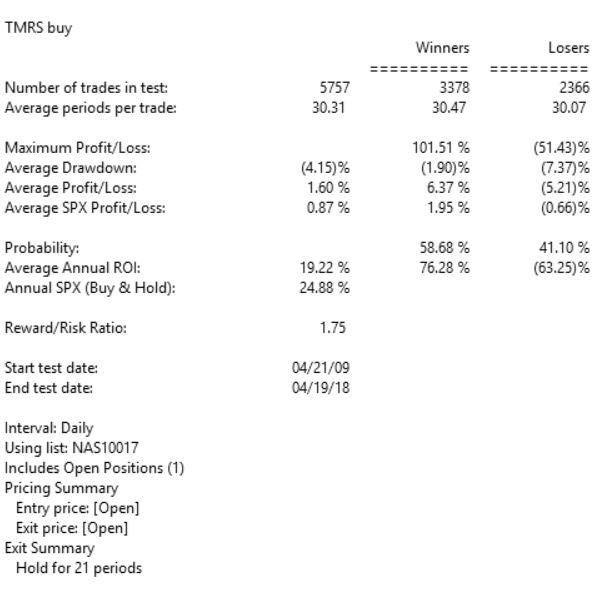FIGURE 11: AIQ, BULL MARKET. Here are the summary results of a backtest using NASDAQ 100 stocks during a generally bullish period from April 2009 to April 2018.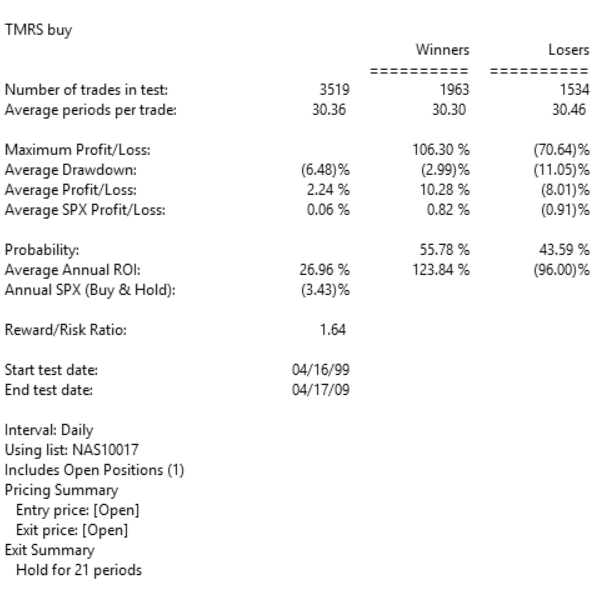FIGURE 12: AIQ, BEAR MARKET. Here are the summary results of a backtest using NASDAQ 100 stocks during a period from April 1999 to April 2009 that contained two bear markets.
—Richard Denning info@TradersEdgeSystems.com for AIQ Systems

# Weekly & Daily Percentage Price Oscillator

The AIQ code based on Vitali Apirine’s article in Stocks & Commodities issue, “Weekly & Daily Percentage Price Oscillator,”  Modifying a traditional indicator can make you look at a chart differently. You can compare indexes, look at price movements during extended periods of time, and make trading decisions based on your observations is provided here:

```!WEEKLY & DAILY PPO
!Author: Vitali Apirine, TASC Feb 2018
!Coded by: Richard Denning 12/17/17

!INPUTS:
S is 12.
L is 26.

EMA1 is expavg([Close],S).
EMA2 is expavg([Close],L).
EMA3 is expavg([Close],S*5).
EMA4 is expavg([Close],L*5).
DM is (EMA1 - EMA2)/EMA4*100.
WM is (EMA3 - EMA4)/EMA4*100.
WD_PPO is WM + DM.```
Figure below shows the daily and weekly PPO indicator on a chart of the Nasdaq 100 index (NDX) from 2015 to 2017.Here, the daily & weekly PPO is displayed on a chart of the NDX.

—Richard Denning info@TradersEdgeSystems.com for AIQ Systems

# Weekly and Daily MACD

The AIQ code based on Vitali Apirine’s article in December 2017 issue of Stocks and Commodities magazine, “Weekly & Daily MACD,” is provided below.
The moving average convergence/divergence oscillator (MACD), developed by Gerald Appel, is one of the more popular technical analysis indicators. The MACD is typically used on a single timeframe, but what if we looked at two timeframes on one chart?

Traders can look for relative daily MACD line crossovers, weekly and daily centerline crossovers, and divergences to generate trading signals.
Figure 5 shows the daily & weekly MACD indicator on a chart of Apple Inc. (AAPL) during 2016 and 2017, when there was a change from a downtrend to an uptrend.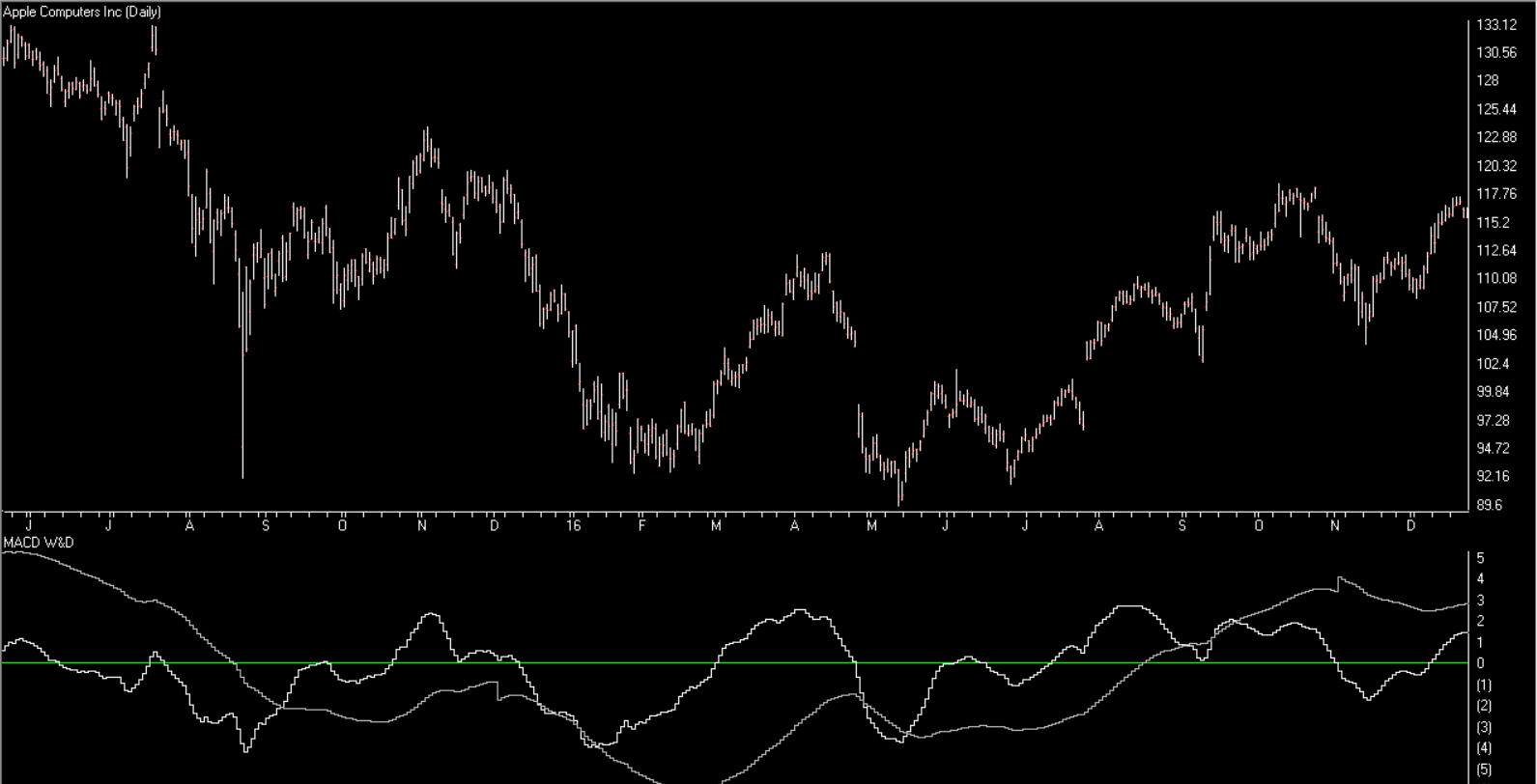FIGURE 5: AIQ. Here is an example of the daily & weekly MACD on a chart of AAPL.
The code and EDS file can be downloaded from http://aiqsystems.com/dailyweeklyMACD.EDS, or copied here:
```!WEEKLY & DAILY MACD
!Author: Vitali Apirine, TASC Dec 2017
!Coded by: Richard Denning 10/13/17

!INPUTS:
S is 12.
L is 25.

EMA1 is expavg([Close],S).
EMA2 is expavg([Close],L).
EMA3 is expavg([Close],S*5).
EMA4 is expavg([Close],L*5).
MACD is EMA1 - EMA2.
MACDW is EMA3 - EMA4.
rdMACD is MACD + MACDW.
```
—Richard Denning
for AIQ Systems

# System Development Using Artificial Intelligence

The AIQ code based on Domenico D’Errico and Giovanni Trombetta’s article in August 2017 Stock & Commodities issue, “System Development Using Artificial Intelligence,” is shown here. You can also download the EDS file from here

Are humans or computers better at trading? This question has been around on many fronts since the era of punch cards, and as technology advances, you question whether machines have limits. It’s the same with trading, and here’s an algorithm that may shed some light on which performs better…

```!ARTIFICAL INTELLIGENCE FOR SYSTEM DEVELOPMENT
!Authors: Domenico D'Errico & Giovanni Trombetta, TASC August 2017
!Coded by: Richard Denning, 6/08/2017

!INPUTS:
O is [open].
C is [close].
H is [high].
L is [low].
exitBars is 8.
exitBarsP is 6.
enterGap is -0.08.

!CODE:
AvgP is (O+C+H+L)/4.
MedP is (H+L)/2.
MedB is (O+C)/2.

AvgP1 is valresult(AvgP,1).
AvgP2 is valresult(AvgP,2).
AvgP3 is valresult(AvgP,3).

MedP1 is valresult(MedP,1).
MedP2 is valresult(MedP,2).
MedP3 is valresult(MedP,3).
MedP4 is valresult(MedP,4).

MedB1 is valresult(MedB,1).
MedB2 is valresult(MedB,2).
MedB3 is valresult(MedB,3).
MedB4 is valresult(MedB,4).

!ENTRY & EXIT RULESl
Gandalf if
(AvgP1exitBars-1)
or ({position days}>=exitBars-1)
or ({position days}>=exitBarsP-1 and (C-{position entry price}>0)).

EntryPr is min(val([low],1) + enterGap,[open]).

Buy if Gandalf and [low] <= EntryPr.

```
See Figure 10 for how to set up the pricing in a backtest.FIGURE 10: AIQ. This shows the EDS backtest settings for entry pricing.
—Richard Denning
for AIQ Systems

# Detecting Swings

The AIQ code based on Domenico D’Errico’s article in the May 2017 issue of Stoks Commodities, “Detecting Swings,” is provided below.

I tested the author’s four systems using the NASDAQ 100 list of stocks on weekly bars, as did the author, from 3/16/2005 through 3/14/2017. Figure 7 shows the comparative metrics of the four systems using the four-week exit. The results were quite different than the author’s, probably due to a different test portfolio and also a 10-year test period rather than the author’s 20-year period. In addition, my test results show longs only, whereas the author’s results are the average of both the longs and shorts.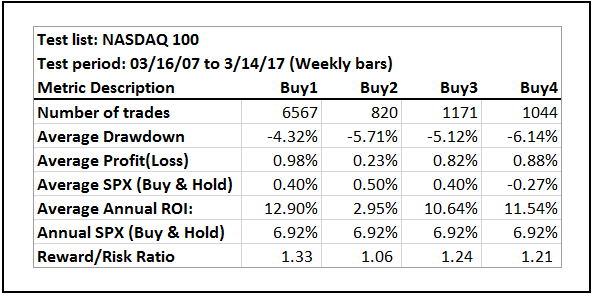FIGURE 7: AIQ. As coded in EDS, this shows the metrics for the author’s four systems run on NASDAQ 100 stocks (weekly bar data) over the period 3/16/2005 to 3/14/2007.

The Bollinger Band (Buy2) system showed the worst results, whereas the author’s results showed the Bollinger Band system as the best. The pivot system (Buy1) showed the best results, whereas the author’s results showed the pivot system as the worst. I am not showing here the comparative test results for the Sell1 thru Sell4 rules, as all showed an average loss over this test period.

```!DECTECTING SWINGS
!Author: Domenico D'Errico, TASC May 2017
!Coded by: Richard Denning, 3/15/17

!Set to WEEKLY in properties

Low is  [low].
Low1  is valresult(Low,1).
Low2  is valresult(Low,2).
High is [high].
High1  is valresult(High,1).
High2  is valresult(High,2).
PivotLow if Low1 < Low2  and Low1 < Low.
PivotHigh if High1 > High2  and High1 > High.

Sell1 if  PivotHigh.

!Set parameter for bollinger bands to 12 with 2 sigma (weekly) in charts:
Buy2 if [close] > [Lower BB] and valrule([close] <= [Lower BB],1).
Sell2 if [close] < [Upper BB] and valrule([close] >= [Upper BB],1).

!Set parameter for Wilder RSI to 5 (weekly) in charts:
Buy3 if [RSI Wilder] > 40 and valrule([RSI Wilder] <= 40,1).
Sell3 if [RSI Wilder] < 60 and valrule([RSI Wilder] >= 60,1).

Buy4 if [RSI Wilder] < 40  And Low > Low1.
Sell4 if [RSI Wilder] > 60  And High < High1.

Exit if {position days} >= 4.```
—Richard Denning
for AIQ Systems
Editor note: The code and EDS file can be downloaded from http://aiqsystems.com/Detecting_Swings_TASC_May_2017.EDS

# Exponential Standard Deviation Bands

The AIQ code based on Vitali Apirine’s article in the 2017 issue of Stocks & Commodities magazine, “Exponential Standard Deviation Bands”

Editor note: “Author Vitali Apirine presented a method intended to help traders see volatility while a stock is trending. These bands, while similar to Bollinger Bands, are calculated using exponential moving averages rather than simple moving averages. Like Bollinger Bands, they widen when volatility increases and narrow as volatility decreases. He suggests that the indicator can be used as a confirming indication along with other indicators such as the ADX. Here’s an AIQ Chart with the Upper, Lower and Middle Exponential SD added as custom indicators.”

To compare the exponential bands to Bollinger Bands, I created a trend-following trading system that trades long only according to the following rules:
1. Buy when there is an uptrend and the close crosses over the upper band. An uptrend is in place when the middle band is higher than it was one bar ago.
2. Sell when the low is less than the lower band.
Figure 8 shows the summary test results for taking all signals from the Bollinger Band system run on NASDAQ 100 stocks over the period 12/9/2000 to 12/09/2016. Figure 9 shows the summary test results for taking all signals from the exponential band system on NASDAQ 100 stocks over the same period. The exponential band system improved the average profit per trade while reducing the total number of trades.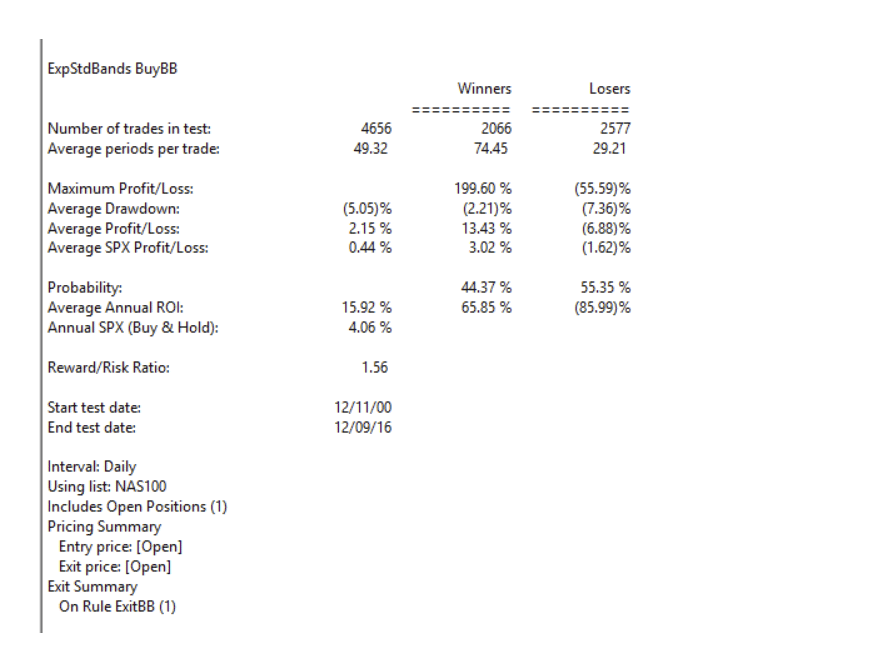FIGURE 8: AIQ. Here are summary test results for taking all signals from the Bollinger Band system run on NASDAQ 100 stocks over the period 12/9/2000 to 12/09/2016.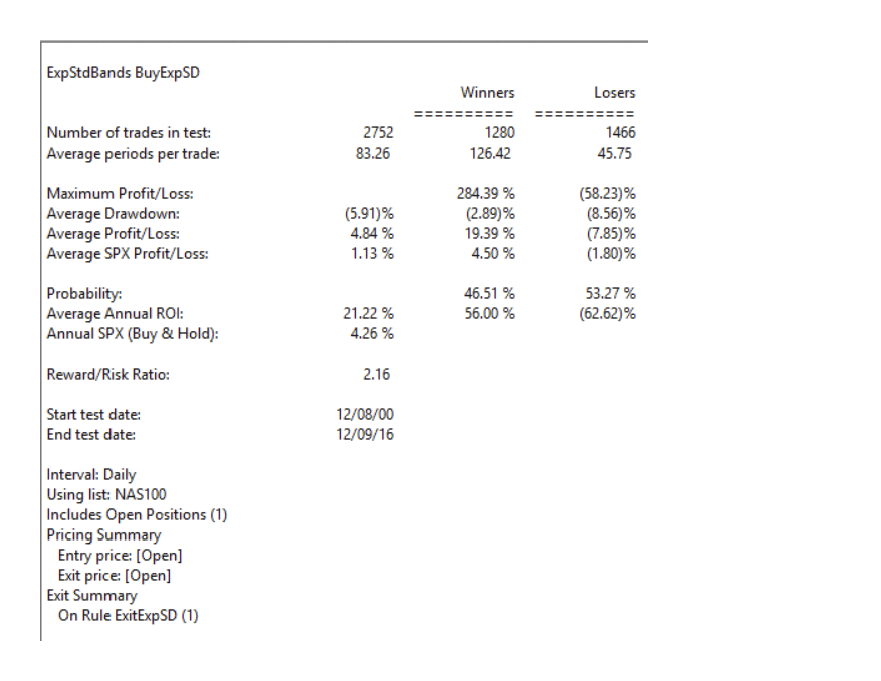FIGURE 9: AIQ. Here are summary test results for taking all signals from the exponential band system run on NASDAQ 100 stocks over the period 12/9/2000 to 12/09/2016.
and is also shown here:
!Exponential Standard Deviation Bands
!Author: Vitali Apirine, TASC February 2017
!Coded by: Richard Denning 12/11/2016
xlen is 20.
numSD is 2.

!INDICATOR CODE:
ExpAvg is expavg([close],xlen).
Dev is [close] – ExpAvg.
DevSqr is Dev*Dev.
SumSqr is sum(DevSqr,xlen).
AvgSumSqr is SumSqr / xlen.
ExpSD is sqrt(AvgSumSqr).

!UPPER EXPONENTIAL SD BAND:
UpExpSD is ExpAvg + numSD*ExpSD.  !PLOT ON CHART

!LOWER EXPONENTIAL SD BAND:
DnExpSD is ExpAvg – numSD*ExpSD.   !PLOT ON CHART

!MIDDLE EXPONENTIAL SD BAND:
MidExpSD is ExpAvg.

!BOLLINGER BANDS FOR COMPARISON:
DnBB is [Lower BB].  !Lower Bollinger Band
UpBB is [Upper BB].  !Upper Bollinger Band
MidBB is simpleavg([close],xlen). !Middle Bollinger Band
!REPORT RULE TO DISPLAY VALUES:
ShowValures if 1.

!TRADING SYSTEM USING EXPPONENTIAL SD BANDS:
UpTrend if MidExpSD > valresult(MidExpSD,1).
BreakUp if [close] > UpExpSD.
BuyExpSD if UpTrend and BreakUp and valrule(Breakup=0,1).
ExitExpSD if [Low] < DnExpSD.  ! or UpTrend=0.